# A bottle

A bottle full of cola weighs 1,320 g. If we drink three-tenths of it, it will weigh 1,008g. How much does an empty bottle weigh?

f =  280 g

### Step-by-step explanation:

f + v = 1320
f + v - 3/10•v = 1008

f+v = 1320
10f+7v = 10080

f = 280
v = 1040

Our linear equations calculator calculates it.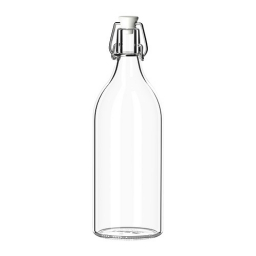Did you find an error or inaccuracy? Feel free to write us. Thank you!Tips to related online calculators
Do you have a linear equation or system of equations and looking for its solution? Or do you have a quadratic equation?
Tip: Our volume units converter will help you with the conversion of volume units.

## Related math problems and questions:

• Jam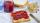Jar with jam weighs 800 grams. Jam weighs three times as much as the empty glass. What is the weight of jam that is in the glass?
• Bag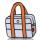Nelly found interesting point. An empty bag weighs 4 kg less than full. And empty bag is 5 times easier than full. How many kg weigh things in this bag?
• BucketThe bucket half filled with water weighs 5.55 kg, the full bucket weighs 9.85 kg. How much does the bucket weigh?
• Bottle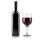Bottle with wine costs 58 USD. Wine is 27 USD more expensive than the empty bottle. How much is the bottle itself?
• Can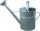Watering can full of water weighs 10 kg. Half-full can weighs 5.5 kg. How much weigh can?
• The barrel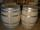The barrel is 135 cm high. If we fill it to a height of 60 cm and it weighs 163 kg. If we fill it to a height of 80 cm, it weighs 213 kg. How many kg weighs a full and how much empty barrel?
• WineA bottle of wine costs 21 euros; and wine is 20 times more expensive than a bottle. How much a bottle cost?
• The devils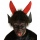The devils weighed in hell with Dorota. They found that Dorota and the two devils weigh 250 kg together, and Dorota and the four devils weigh 426 kg. All the devils weigh the same. How Much Does Dorota Weigh?
• Conical bottleWhen a conical bottle rests on its flat base, the water in the bottle is 8 cm from it vertex. When the same conical bottle is turned upside down, the water level is 2 cm from its base. What is the height of the bottle?
• Barrel of oilBarrel of oil weighs 283 kg. When it mold 26% oil, weighed 216 kg. What is the mass of the empty barrel?
• Father and sonFather and son weigh together 108kg. The father weighs 2.6 times more than his son. How much does weight my father and son count?
• Barrel 4Barrel of water weighs 63 kg. After off 75% water, the weight of the barrel with water is 21 kg. How many kg weigh empty barrel and how many kgs water in it?
• Watering can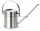A watering can full of water weighs 11 kg, the weight of water is 10 kg greater than the weight of an empty watering can, how much is the weight of the watering can?
• The cube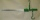The cube and cylinder weigh the same as three 20-gram weights. Three cubes and one cylinder weigh the same as two cylinders and one cube. How many grams does one cube weigh (and one cylinder)?
• The water barrel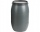The water barrel weighs 122 kg. If we pour 75% of the water out of it, it will weigh 35 kg. What is the weight of the barrel?
• Children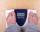Margaret and Zdena weigh the same and Petra 3 kg more. Together weigh 156 kg. How much do they weigh?
• Water containerContainer with water weighs 1.48 kg. When we cast 75% of water container of water weight 0.73 kg. How heavy is an empty container?